# How to remember

## Annual interest formula remember

The formula is:

A=P(1+r/n)^(nt)

To remember this thinkThe annual that was most interesting in my childhood:Annual of most interest had a front cover that looked like a face.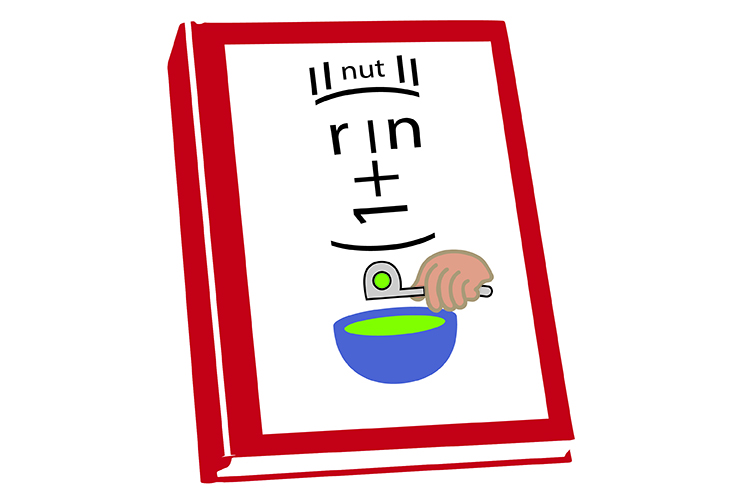Draw this interesting face and you won't forget this formula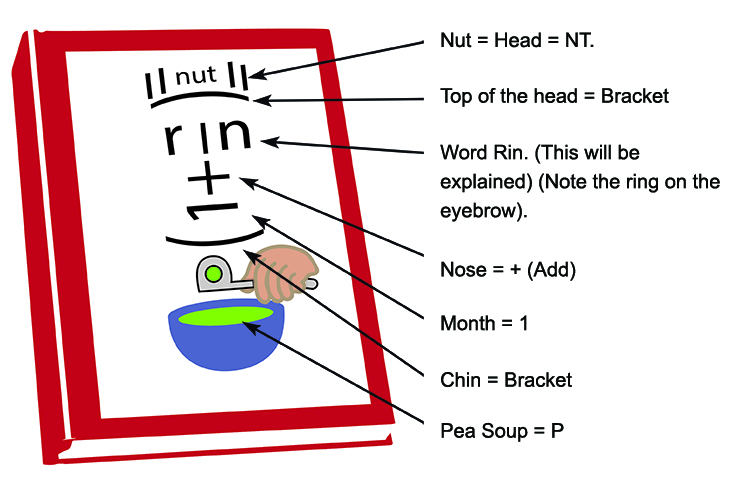Note:

Rin nearly spells ring, which many people have in their nose or eyebrows.

P is eating Pea soup.

Now turn this sideways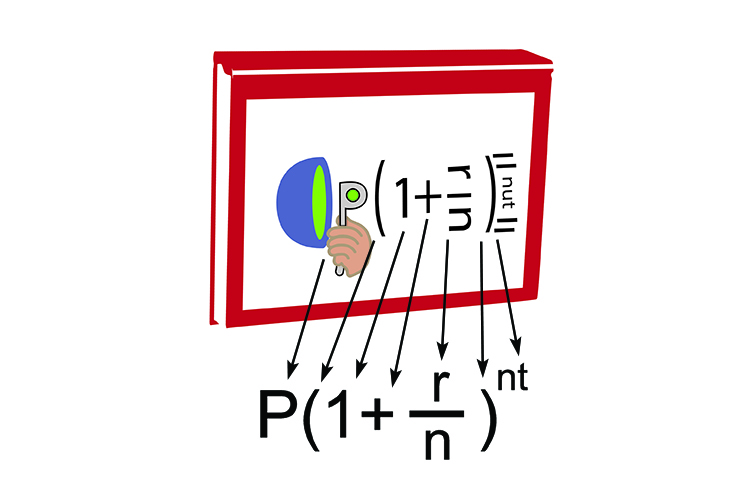Do a quick sketch – Then rotate it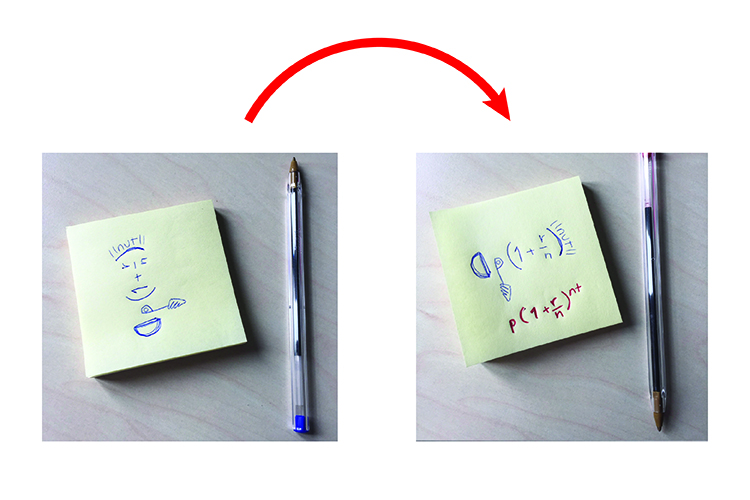## Formula symbol meaning

A=P(1+r/n)^(nt)

How do you know what each symbol means?

From our picture:

1.   Annual book that was most interesting.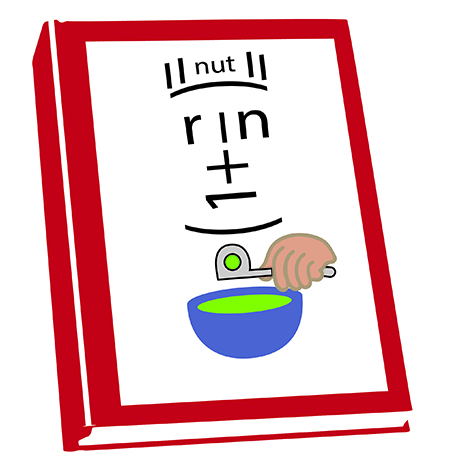A = Annual interest formula

But the A also stands for ALL as in all the total interest.

2.  Pea green soup (Principal).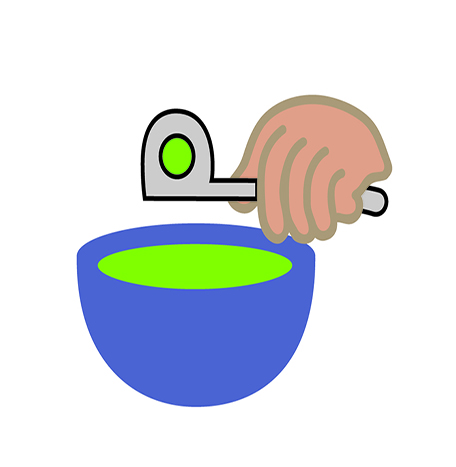P = Principal (starting amount)

3.  r can only be rate

r = rate of interest

(What else could it be?)

4.  t = time (in years)

(What else could it be?)

5.  n = If n stands for night time, there are 365 nights in a year. So if the interest compounded every night n would be 365.

n = number of compounds in a year.